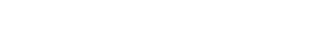# The influence of cables on the parameters of the speaker

Created on 01/22/2015 10:20 AM.

Updated 02.02.2020 19:20.

Author: Sigizmund_III.

The overwhelming majority of audiophiles are convinced of the significant effect of cables on sound in speakers. Many articles have been written on this topic, both supporters and opponents of this theory, however, I have not come across a single article containing real technical calculations that would prove this or that point of view. The texts usually contain their own conjectures, which are sometimes far from reality. I used technical knowledge and calculations to understand this topic.

Most often, in addition to the active resistance of the conductor, audiophiles mention three factors supposedly affecting the final parameters of the electrical circuit:

• capacitive resistance (since the cable consists of a pair of conductors);
• inductive resistance;
• skin effect.

Let’s look at the first two factors together, as they are very closely related.

The fact is that there is an equivalent circuit of an infinitely small segment of a long power line, which is a four-pole device containing linear resistance, capacitance, inductance and conductivity (Figure 1). Thus, any long line is a collection of data of four-port networks connected in series.Figure 1 – Equivalent circuit of an infinitely small segment of a long line

However, here it should be borne in mind that we are talking about a long line. By definition, a long line is a regular power line, the length of which is many times the wavelength of oscillations propagating in it, and the distance between the conductors and the transverse size of the conductors is many times less than the wavelength, i.e. the relations holdwhere λ – wavelength, L – line length, a – conductor cross-section, b – the distance between the conductors. For the upper cutoff frequency ν = 20000 Hz audible wavelength λ = c⁄ν, where c – the speed of light will be equal to 300,000,000 / 20,000 = 15,000 m, or 15 km. For a frequency of 50 Hz, the wavelength will reach six thousand kilometers. Naturally, these lengths of speaker cables are not used, and therefore the long line model is clearly not suitable for them.

For lines, the length of which is much shorter or commensurate with the wavelength of oscillations, there is an equivalent circuit of a short line (Figure 2).Figure 2 – Equivalent diagram of an infinitely small segment of a short line.

As can be seen from the figure, the line conductivity and inductance are no longer taken into account here, since their values ​​are negligible (for a short line). This means that there is no point in considering the second factor. Only the capacity remains.

Let us now calculate the input and output resistance of our passive four-port network and see its transfer characteristic. The input impedance for the first circuit will be equal to:Output impedance for the second circuit:Voltage transfer characteristic:Transfer characteristic module:Now let’s take one meter of some real cable for calculation. I went to audiomania.ru and found a cheap Onetech Rapid Two INT0107 microphone cable. One conductor of such a cable has a cross section of 0.21 mm2, which roughly corresponds to the AWG 24 gauge, according to the American standard. From the book Fundamentals of Telecommunications, we will use the table in which the linear resistances and capacitances are indicated (Figure 3).Figure 3 – Table of cable parameters (for 1 kHz).

For AWG 24 C = 40 nF⁄km= 40 pF⁄m; R = 170 Om⁄km= 0.17 Om⁄m,ν = 1000 Hz. Substitute these values ​​into formula (4):I deliberately left more than 15 decimal places to show how scanty the voltage change is when passing through a four-pole. By the way, it is difficult even to find a device that will show such accuracy.

Let’s now look at the boundary value of the spectrum of frequencies perceived by the human ear (ν_n = 20 Hz, ν_w = 20000 Hz):Skeptics will say: “This is just a calculation for one meter of cable.” Well, let’s see what happens to the voltage transfer characteristic module for, say, five meters of cable (for 1 kHz).The changes for five meters of cable are still negligible to account for.

By definition, the skin effect (or surface effect) is the effect of decreasing the amplitude of electromagnetic waves as they penetrate deep into the conductive medium. As a result of this effect, for example, an alternating current of high frequency, when flowing through a conductor, is not evenly distributed over the section, but mainly in the surface layer. It is due to the uneven distribution of the current that the effective cross-section of the conductor decreases, and, consequently, the resistance increases.

This idea of ​​skin effect makes audiophiles buy silver-plated wires, which, of course, are much more expensive than conventional wires (with a thin layer of silver it is really possible to combat skin effect at high frequencies, due to the lower resistivity of silver). But does it make sense?

The derivation of the formula describing the skin effect is based on the Maxwell equation. It makes no sense to describe it, all the information can be found in textbooks for universities (for example, in the textbook of Sivukhin). Instead of a conclusion, we will use a simplified formula for calculating the thickness of the skin layer (the layer in the conductor where almost all the current is concentrated):where ρ – resistivity, μ_m – relative magnetic permeability, f – frequency.

For copper: ρ = 0.018 (Ohm ∙ sq.mm) / m; μ_m = 0.999994 at frequency f = 20,000 Hz:Let’s calculate the cross-sectional area in which we observe the skin effect:Thus, for any wire gauge that has a cross-sectional area less than 2.95 sq. Mm, the skin effect has no effect at all.